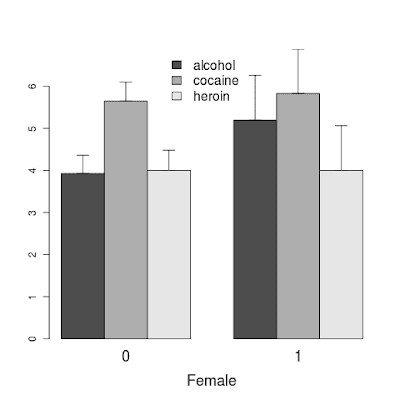## Tuesday, November 22, 2011

### Example 9.15: Bar chart with error bars ("Dynamite plot")The "dynamite plot", a bar chart plotting the a mean with a error bar, is one of the most reviled types of image among statisticians. Reasons to dislike them are numerous, and are nicely summarized here. (Edward Tufte also suggests they be avoided.)

Nonetheless, as consulting statisticians, we're often required to meet the needs of our collaborators, or of the reviewers who will judge their submissions. If you need to do it, here's how. We demonstrate with the HELP data set.

SAS

The plot can be created easily with proc gchart (section 5.1.3).
`proc gchart data = "c:\book\help.sas7bdat";vbar substance /   group=female   type=mean    sumvar=sexrisk   errorbar=top;run;`

The syntax requests the basic chart variable be substance, in groups defined by female, where the mean of sexrisk is plotted. The errorbar option can be used to make the full CI or just the top lines; the default is a 95% standard error of the mean, but that can also be adjusted. The result is shown below.R

There are several easily googlable examples of how to do a basic dynamite plot in R, including one in example(barchart). However, a grouped example with a nice legend is a bit harder to find. The bargraph.CI() function in the sciplot package fits the bill.
`library(sciplot)bp = with(ds, bargraph.CI(x.factor=female, group=substance, response=sexrisk,  lc=FALSE, xlab="Female",  legend=TRUE, x.leg=3.3, cex.leg=1.3, cex.names=1.5, cex.lab = 1.5,  ci.fun=function(x) {c(mean(x) - 1.96*se(x), mean(x) + 1.96*se(x))}))`

The results are shown at the top. The first row of options describe the variables to be used; the default plot statistic is the mean. The second and third rows suppress the bottom of the confidence interval and customize the location, label, and font size in the legend and the x-axis label. The only tricky bit is the last row, which provides the 95% CI limits to be plotted, as opposed to the default 1 standard error.

#### 1 comment:

Rick Wicklin said...

For more on this topic, see http://blogs.sas.com/content/iml/2011/10/07/creating-bar-charts-with-confidence-intervals/